# Potential energyThe cars of a roller coaster reach a maximum of gravitational potential energy when at a crest along their path. When they start moving downward, the potential energy begins to be converted to kinetic energy. The sum of kinetic and potential energy in the system remains constant, assuming negligible losses due to friction.

Potential energy can be thought of as energy stored within a physical system. It is called potential energy because it has the potential to be converted into other forms of energy, particularly kinetic energy, and to do work in the process. The standard (SI) unit of measure for potential energy is the joule, the same as for work or energy in general.

There are various types of potential energy, each associated with a particular type of force. They include elastic potential energy, gravitational potential energy, electric potential energy, nuclear potential energy, intermolecular potential energy, and chemical potential energy.

## Overview

The term "potential energy" was coined by William Rankine, a nineteenth-century Scottish engineer and physicist. It corresponds to energy that is stored within a system. It exists when there is a force that tends to pull an object back towards some original position when the object is displaced. This force is often called a restoring force.

For example, when a spring is stretched to the left, it exerts a force to the right so as to return to its original, unstretched position. Similarly, when a weight is lifted up, the force of gravity will try to bring it back down to its original position. The initial steps of stretching the spring or lifting the weight both require energy to perform. According to the principle of conservation of energy, energy cannot be created or destroyed; hence this energy cannot disappear. Instead, it is stored as potential energy. If the spring is released or the weight is dropped, this stored energy will be converted into kinetic energy by the restoring force—elasticity in the case of the spring, and gravity in the case of the weight.

The more formal definition is that potential energy of a system is the energy of position, that is, the energy a system is considered to have due to the positions of its components in space. For given positions of all other objects of the system, the potential energy is a function of the position of a given object.

There are a number of different types of potential energy, each associated with a particular type of force. More specifically, every conservative force gives rise to potential energy. For example, the work of elastic force is called elastic potential energy; work of gravitational force is called gravitational potential energy, work of the Coulomb force is called electric potential energy; work of strong nuclear force or weak nuclear force acting on the baryon charge is called nuclear potential energy; work of intermolecular forces is called intermolecular potential energy. Chemical potential energy, such as the energy stored in fossil fuels, is the work of the Coulomb force during rearrangement of mutual positions of electrons and nuclei in atoms and molecules. Thermal energy usually has two components: the kinetic energy of random motion of particles and potential energy of their mutual positions.

As a general rule, the work done by a conservative force F will be$\,W = -\Delta U$

where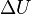$\Delta U$ is the change in the potential energy associated with that particular force. The most common notations for potential energy are PE and U. Electric potential (commonly denoted with a V for voltage) is the electric potential energy per unit charge.

## Chemical potential energy

Chemical potential energy is a form of potential energy related to the structural arrangement of atoms or molecules. This arrangement may be the result of chemical bonds within a molecule or otherwise. Chemical energy of a chemical substance can be transformed to other forms of energy by a chemical reaction. For example, when a fuel is burned the chemical energy is converted to heat, same is the case with digestion of food metabolized in a biological organism. Green plants transform solar energy to chemical energy through the process known as photosynthesis, and electrical energy can be converted to chemical energy through electrochemical reactions.

The similar term chemical potential is used by chemists to indicate the potential of a substance to undergo a chemical reaction.

## Electrical potential energy

An object can have potential energy by virtue of its electric charge and several forces related to their presence. There are three main types of this kind of potential energy: electrostatic potential energy, electrodynamic potential energy (also sometimes called magnetic potential energy), and nuclear potential energy.

### Electrostatic potential energy

In case the electric charge of an object can be assumed to be at rest, it has potential energy due to its position relative to other charged objects.

The electrostatic potential energy is the energy of an electrically charged particle (at rest) in an electric field. It is defined as the work that must be done to move it from an infinite distance away to its present location, in the absence of any non-electrical forces on the object. This energy is non-zero if there is another electrically charged object nearby.

The simplest example is the case of two point-like objects A1 and A2 with electrical charges q1 and q2. The work W required to move A1 from an infinite distance to a distance d away from A2 is given by:$W=k\frac {q_1q_2} d$

where k is Coulomb's constant, equal to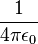$\frac 1 {4\pi\epsilon_0}$.

This equation is obtained by integrating the Coulomb force between the limits of infinity and d.

A related quantity called electric potential is equal to electric potential energy of a unit charge.

### Electrodynamic potential energy

In case a charged object or its constituent charged particles are not at rest, it generates a magnetic field giving rise to yet another form of potential energy, often termed as magnetic potential energy. This kind of potential energy is a result of the phenomenon magnetism, whereby an object that is magnetic has the potential to move other similar objects. Magnetic objects are said to have some magnetic moment. Magnetic fields and their effects are best studied under electrodynamics.

## Nuclear potential energy

Nuclear potential energy is the potential energy of the particles inside an atomic nucleus, some of which are indeed electrically charged. This kind of potential energy is different from the previous two kinds of electrical potential energies because in this case the charged particles are extremely close to each other. The nuclear particles are bound together not because of the coulombic force, but due to strong nuclear force that binds nuclear particles more strongly and closely. Weak nuclear forces prepare the potential energy for certain kinds of radioactive decay, such as beta decay.

Nuclear particles like protons and neutrons are not destroyed in fission and fusion processes, but collections of them have less mass than if they were individually free, and this mass difference is liberated as heat and radiation in nuclear reactions (the heat and radiation have the missing mass, but it often escapes from the system, where it is not measured). The energy from the Sun, also called solar energy, is an example of this form of energy conversion. In the Sun, the process of hydrogen fusion converts about 4 million metric tons of solar matter per second into light, which is radiated into space.

## Thermal potential energy

The thermal energy of an object is simply the sum of the kinetic energies of the particles constituting it (which are in random motion) plus the potential energies of their displacements from their equilibrium positions as they oscillate or move around them. In the case of an ideal gas, there is no potential energy due to interactions of particles, but kinetic energy may include a rotational part too (for multiatomic gases)—if rotational levels are excited at a given temperature T.

Solar updraft towers use this kind of power.

## Rest mass energy

Albert Einstein was the first to calculate the amount of work needed to accelerate a body from rest to some finite speed using his definition of relativistic momentum. To his surprise, this work contained an extra term that did not vanish as the speed of the accelerated body approached zero: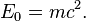$E_0 = m c^2. \,$

This term (E0) was therefore called rest mass energy, as m is the rest mass of the body (c is the speed of light which is constant) (The subscript zero is used here to distinguish this form of energy from the others that follow. In most other contexts, the equation is written with no subscript.)

So, the rest mass energy is the amount of energy inherent in the mass when it is at rest. If the mass changes, so must its rest mass energy which must be released or absorbed due to energy conservation law. Thus, this equation quantifies the equivalence of mass and energy.

Due to the large numerical value of squared speed of light, even a small amount of mass is equivalent to a very large amount of energy, namely 90 petajoules per kilogram ≈ 21 megaton of TNT per kilogram.

## Relation between potential energy and force

Potential energy is closely linked with forces. If the work done moving along a path which starts and ends in the same location is zero, then the force is said to be conservative and it is possible to define a numerical value of potential associated with every point in space. A force field can be re-obtained by taking the vector gradient of the potential field.

For example, gravity is a conservative force. The work done by a unit mass going from point A with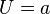$U = a$ to point B with$U = b$ by gravity is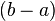$(b - a)$ and the work done going back the other way is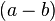$(a - b)$ so that the total work done from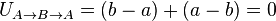$U_{A \to B \to A} = (b - a) + (a - b) = 0 \,$

If we redefine the potential at A to be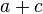$a + c$ and the potential at B to be$b + c$ [where$c$ can be any number, positive or negative, but it must be the same number for all points] then the work done going from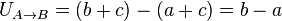$U_{A \to B} = (b + c) - (a + c) = b - a \,$

as before.

In practical terms, this means that you can set the zero of$U$ anywhere you like. You might set it to be zero at the surface of the Earth or you might find it more convenient to set it to zero at infinity.

A thing to note about conservative forces is that the work done going from A to B does not depend on the route taken. If it did, then it would be pointless to define a potential at each point in space. An example of a non-conservative force is friction. With friction, the route you take does affect the amount of work done, and it makes no sense at all to define a potential associated with friction.

All the examples above are actually force-field-stored energy (sometimes in disguise). For example in elastic potential energy, stretching an elastic material forces the atoms very slightly further apart. The equilibrium between electromagnetic forces and Pauli repulsion of electrons (they are fermions obeying Fermi statistics) is slightly violated resulting in a small returning force. Scientists rarely talk about forces on an atomic scale. Often interactions are described in terms of energy rather than force. You can think of potential energy as being derived from force or you can think of force as being derived from potential energy (though the latter approach requires a definition of energy that is independent from force which does not currently exist).

A conservative force can be expressed in the language of differential geometry as a closed form. Because Euclidean space is contractible, its de Rham cohomology vanishes, so every closed form is exact, i.e., is the gradient of a scalar field. This gives a mathematical justification of the fact that all conservative forces are gradients of a potential field.